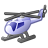# 4.19 UniqueValueRenderer.removeUniqueValueInfo Bug

200
1
06-30-2021 02:34 PMOccasional Contributor III

The value property of a UniqueValueInfo instance can be either a String or a Number, as documented.  Therefore, the documentation also indicates that the removeUniqueValueInfo method of the UniqueValueRenderer class accepts either a String or a Number.  However, this method will not remove UniqueValueInfo instances if their value property has a numeric value, regardless of whether you pass a String or a Number to the function.

The reason for this can be seen inside the definition of the removeUniqueValueInfo function:

``````for (let b = 0; b < this.uniqueValueInfos.length; b++)
if (this.uniqueValueInfos[b].value === a + "") {
delete this._valueInfoMap[a];
this.uniqueValueInfos.splice(b, 1);
break
}``````

In the "if" statement of line 2 above, the variable "a" contains the value passed into the function.  In this same line, if the left-hand side of the comparison is a Number, then the result will always be false, because the identity/strictly equals operator (===) is used, and the right-hand side will always be a String.

My fix for 4.19 is to make the following change in the init.js file (assuming a locally hosted API):

Search For:

``if(this.uniqueValueInfos[N].value===S+"")``

Replace With:

``if(this.uniqueValueInfos[N].value+""===S+"")``

Note, I've verified the same problem goes back at least as far as 4.16, but this fix is specific to 4.19.by